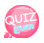# Variable declaration

So far I have used \$ ('SELECTOR'); when selecting id, class or tag.

Every time you declare a selector, the computer checks the html source, and if so, it checks again and repeats this task.

But if you declare it as a variable, it is loaded only once when you first declare it and the information is stored in the variable.

Doing things as simple and short as you do today will hardly speed you up, but it can slow your site down when large sources are used.

So let's declare a variable. You declare a variable with var, like JavaScript.

Let's assume that we have a class called hello. Let's declare this hello class.

`\$('.hello');`

In the past, I declared something like the above and attached it to some function.

But if you declare a variable

`var hello = \$('.hello');`

var is the command used to declare variables. hello is a name that you declare arbitrarily. And hello means \$ ('.hello'); It means.

How do you use it?

```var hello = \$('.hello');
hello.hide(); ex) If you use a variable without declaring it -> \$('.hello').hide();
hello.show(); ex)If you use a variable without declaring it -> \$('.hello').show();```

In other words, it is equivalent to writing \$ ('.hello') by creating a variable called hello.

So let's practice by example using variables.

```<!DOCTYPE html>
<html>
<meta charset="utf-8" />
<title>jQuery</title>
<style>
</style>
<script type="text/javascript" src="https://code.jquery.com/jquery-3.2.0.min.js" ></script>
<script type="text/javascript">
\$(function(){
var yellow_circle = \$('.yellow_circle');
var yellow_circle_word = \$('.yellow_circle_word');

yellow_circle.mouseenter(function(){
yellow_circle_word.text('The mouse pointer is in a yellow circle.');
});

yellow_circle.mouseleave(function(){
yellow_circle_word.text('The mouse pointer has left the yellow circle.');
});
});
</script>
<body>
<div class="yellow_circle"></div>
<p class="yellow_circle_word">test is</p>
</body>
</html>```

Result of Example Using Variables

test it

#### Learn Variables with Another Example

You can also display an alert window using jQuery. ^^

```<!doctype html>
<html>
<meta charset="utf-8" />
<title>jQuery</title>
<script type="text/javascript" src="https://code.jquery.com/jquery-3.2.0.min.js" ></script>
<script type="text/javascript">
\$(function(){
var usa_hi = 'hi';
var jp_hi = 'konnichiha';
var kr_hi = 'annyeong';
var cn_hi = 'nihao';
var con1 = usa_hi + jp_hi;
var con2 = con1 + kr_hi;
});
</script>
<body>
</body>
</html>```

You can also use as above.

If you put usa_hi in parentheses in alert() ;, the value hi of usa_hi is output.

And in alert() if usa_hi is wrapped with ''

`alert('usa_hi');`

If you input as above, the phrase usa_hi will be printed instead of hi. If you wrap '', the phrase comes out. If there is no '', it is recognized as a variable.

`var con1 = usa_hi + jp_hi;`

This means that the value hi of usa_hi and the value konnnichiha of jp_hi are combined to enter the value of con1.

So the value of con1 would be hikonnnichiha? ^^

end.. Thank you ^^